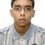# Probability

A person writes 4 letters & addresses 4 envelops randomly. What is the probability that every letters goes to the wrong address?Note by Maharnab Purkait
6 years, 9 months ago

This discussion board is a place to discuss our Daily Challenges and the math and science related to those challenges. Explanations are more than just a solution — they should explain the steps and thinking strategies that you used to obtain the solution. Comments should further the discussion of math and science.

When posting on Brilliant:

• Use the emojis to react to an explanation, whether you're congratulating a job well done , or just really confused .
• Ask specific questions about the challenge or the steps in somebody's explanation. Well-posed questions can add a lot to the discussion, but posting "I don't understand!" doesn't help anyone.
• Try to contribute something new to the discussion, whether it is an extension, generalization or other idea related to the challenge.

MarkdownAppears as
*italics* or _italics_ italics
**bold** or __bold__ bold
- bulleted- list
• bulleted
• list
1. numbered2. list
1. numbered
2. list
Note: you must add a full line of space before and after lists for them to show up correctly
paragraph 1paragraph 2

paragraph 1

paragraph 2

[example link](https://brilliant.org)example link
> This is a quote
This is a quote
    # I indented these lines
# 4 spaces, and now they show
# up as a code block.

print "hello world"
# I indented these lines
# 4 spaces, and now they show
# up as a code block.

print "hello world"
MathAppears as
Remember to wrap math in $$ ... $$ or $ ... $ to ensure proper formatting.
2 \times 3 $2 \times 3$
2^{34} $2^{34}$
a_{i-1} $a_{i-1}$
\frac{2}{3} $\frac{2}{3}$
\sqrt{2} $\sqrt{2}$
\sum_{i=1}^3 $\sum_{i=1}^3$
\sin \theta $\sin \theta$
\boxed{123} $\boxed{123}$

Sort by:

The answer is $\displaystyle \frac{9}{24}$ or $\displaystyle \frac38$

I am not going details. Just note that this is called Derangement .Try the link and reply if anything seems difficult.

- 6 years, 9 months ago

Thanks, please give me details calculation

- 6 years, 9 months ago

Sadman is correct. The way I tackled it is by thinking of just as ways I can arrange A, B, C and D without having any letters match the column that they're in (A, B, etc.). If you just write on your paper ABCD, then you can simply list them all out. Here they are: BADC BDAC BCDA CDBA CADB CDAB DCBA DCAB DABC You'll notice that each letter is used 3 times in each column. That is because that's how many wrong addresses there are, multiplied by 3 for the number of total ways. The numerator is 9, therefore, and the denominator is 432*1 which equals 24. The answer is 9/24 or 3/8.

- 6 years, 9 months ago

A person writes 4 letters & addresses 4 envelops randomly. What is the probability that every letters goes to the wrong address?

- 6 years, 9 months ago

1/4

- 6 years, 9 months ago

Three winning tickets are drawn from an urn of - 100 tickets . what is the probability of winning for a person who buys - (i) 4 tickets ? (ii) only one tickets?

- 6 years, 9 months ago

to me the answer is 1/4 ......

Calculation details:

for first letter , since there are 4 envelopes, it can go to right address in only one case.... and wrong in three cases, so the probability of going to wrong address is 3/4

for 2nd letter , since there are 3 envelopes left now,it can go to right address in only one case.... and wrong in two cases, so for 2nd letter the probability of going to wrong address is 2/3

for 3rd letter , since there are 2 envelopes left now,it can go to right address in only one case.... and wrong in one case, so the probability of going to wrong address is 1/2

for 4th letter , since there is 1 envelope left and we have been assuming that others have gone to wrong addresses , so it must go to the wrong address. so its probability of going to wrong address is 1.

multiplying all we get, 3/4 * 2/3 * 1/2 * 1 = 1/4

(here multiplication is done, since we consider all of them are going to wrong addresses i.e simplifying we can write....

probability of (first letter will go to wrong address and 2nd letter will go to wrong address and 3rd letter will go to wrong address and 4th letter will go to wrong address and )

i.e probability of (first letter will go to wrong address * 2nd letter will go to wrong address *
3rd letter will go to wrong address * 4th letter will go to wrong address and )

therefore, P= ( 3/4 * 2/3 * 1/2 * 1 ) = 1/4.

so, the answer is 1/4 . )

- 6 years, 9 months ago

Answer is 9/24.You are making a big mistake in the second step,Pavel. Consider a situation; letter no 1 is put in the envelope of any other letter, say in the envelope of letter 2. Now what if letter 2 is considered next. This case letter 2 has 3 choices again.As letter1 is occupying its envelope it can enter in any remaining envelope.Got it? Sadman is correct. This can only be solved using derangement formula .You can see it in any combinatorics books.It can be proved using logic of inclusion-exclusion of set theory.

- 6 years, 9 months ago

yeah i got it..... thnqu :)

- 6 years, 9 months ago